### MEMO 2018 ekipno problem 5

Kvaliteta:
Avg: 0,0
Težina:
Avg: 6,0

Let$ABC$ be an acute-angled triangle with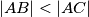$|AB| < |AC|$, and let$D$ be the foot of its altitude from$A$. Points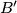$B'$ and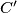$C'$ lie on the rays$AB$,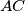$AC$ respectively, so that points$B'$,$C'$ and$D$ are collinear and points$B$,$C$,$B'$,$C'$ are concyclic with center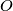$O$. Prove that if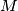$M$ is the midpoint of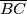$\overline{BC}$ and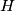$H$ is the orthocenter of$ABC$, then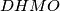$DHMO$ is a parallelogram.

Izvor: Srednjoeuropska matematička olimpijada 2018, ekipno natjecanje, problem 5